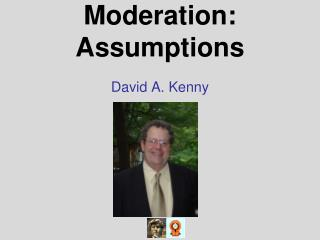DownloadDownload PresentationModeration: Assumptions

# Moderation: Assumptions

Download Presentation## Moderation: Assumptions

- - - - - - - - - - - - - - - - - - - - - - - - - - - E N D - - - - - - - - - - - - - - - - - - - - - - - - - - -
##### Presentation Transcript

1. Moderation: Assumptions David A. Kenny

2. What Are They? Causality Linearity Homogeneity of Variance No Measurement Error

3. Causality • X and M must both cause Y. • Ideally both X and M are manipulated variables and measured before Y. Of course, some moderators cannot be manipulated (e.g., gender).

4. Causal Direction • Need to know causal direction of the X to Y relationship. • As pointed out by Irving Kirsch, direction makes a difference!

5. Surprising Illustration • Judd & Kenny (2010, Handbook of Social Psychology), pp. 121-2 (see Table 4.1). • A dichotomous moderator with categories A and B • The X  Y effect can be stronger for the A’s than the B’s. • The Y  X effect can be stronger for the B’s than the A’s.

6. Direction of Causality Unclear • In some cases, causality is unclear or the two variables may not even be a direct causal relationship. • Should not conduct a moderated regression analysis. • Tests for differences in variances in X and Y, and if no difference, test for differences in correlation.

7. Crazy Idea? • Assume that either X  Y or Y  X. • Given parsimony, moderator effects should be relatively weak. • Pick the causal direction by the one with fewer moderator effects.

8. Proxy Moderator • Say we find that Gender moderates the X  Y relationship. • Is it gender or something correlated with gender: height, social roles, power, or some other variable. • Moderators can suggest possible mediators.

9. Graphing • Helpful to look for violations of linearity and homogeneity of variance assumptions. • M is categorical. • Display the points for M in a scatterplot by different symbols. • See if the gap between M categories change in a nonlinear way.

10. Linearity • Using a product term implies a linear relationship between M and X to Y relationship: linear moderation. • The effect of X on Y changes by a constant amount as M increases or decreases. • It is also assumed that the X  Y effect is linear: linear effect of X.

11. Alternative to Linear Moderation • Threshold model: For X to cause Y, M must be greater (lesser) than a particular value. • The value of M at which the effect of X on Ychanges might be empirically determined by adapting an approach described by Hamaker, Grasman, and Kamphuis (2010).

12. Second Alternative to Linear Moderation • Curvilinear model: As M increases (decreases), the effect of X on Y increases but when M gets to a particular value the effect reverses.

13. Testing Linear Moderation • Add M2 and XM2 to the regression equation. • Test the XM2 coefficient. • If positive, the X  Y effect accelerates as M increases. • If negative, then the X  Y effect de-accelerates as M increases. • If significant, consider a transformation of M.

14. The Linear Effect of X • Graph the data and look for nonlinearities. • Add X2 and X2M to the regression equation. • Test the X2 and X2M coefficients. • If significant, consider a transformation of X.

15. Nonlinearity or Moderation? • Consider a dichotomous moderator in which not much overlap with X (X and M highly correlated). • Can be difficult to disentangle moderation and nonlinearity effects of X.

16. Nonlinear Relationship Y X Moderation Y X

17. Homogeneity of Variance • Variance in Moderation Analysis • X • Y (actually the errors in Y)

18. Different Variance in X for Levels of M • Not a problem if regression coefficients are computed. • Would be a problem if the correlation between X and Y were computed. • Correlations tend to be stronger when more variance.

19. Equal Error Variance • A key assumption of moderated regression. • Visual examination • Plot residuals against the predicted values and against X and Y • Rarely tested • Categorical moderator • Bartlett’s test • Continuous moderator • not so clear how to test

20. Violation of Equal Error Variance Assumption: Categorical Moderator • The category with the smaller variance will have too weak a slope and the category with the larger variance will too strong a slope. • Separately compute slopes for each of the groups, possibly using a multiple groups structural equation model.

21. Violation of Equal Error Variance Assumption: Continuous Moderator • No statistical solution that I am aware of. • Try to transform X or M to create homogeneous variances.

22. Variance Differences as a Form of Moderation • Sometimes what a moderator does is not so much affect the X to Y relationship but rather alters the variances of X and Y. • A moderator may reduce or increase the variance in X. • Stress  Mood varies by work versus home; perhaps effects the same, but much more variance in stress at work than home.

23. Measurement Error • Product Reliability (X and M have a normal distribution) • Reliability of a product: rxrm(1 + rxm2) • Low reliability of the product • Weaker effects and less power • Bias in XM Due to Measurement Error in X and M • Bias Due to Differential X Variance for Different Levels of M

24. Differential Reliability • categorical moderator • differential variances in X • If measurement error in X, then reliability of X varies, biasing the two slopes differentially. • Multiple groups SEM model should be considered

25. Additional Webinars • Effect Size and Power • ModText